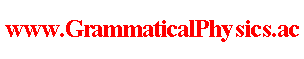since 2006 Help　Sitemap < Forum > < Problems > < Quantum History Theory > < Twist Remove Normalization > I suppose that a general definition of the twist remove normalization for a functional Φ is given by the condition:∫Dχ Φ[χ]* Φ[χ] = 1,(d/dε) ∫Dχ Φ[χ]* Φ[χ(□ - ε)] |ε = 0 = 0,where χ(□ - ε) is a function such that[χ(□ - ε)](t) = χ(t - ε) for all t. Possibly, a general definition of the twist remove normalization for a functional Φ is given by the condition:∫Dχ Φ[χ]* Φ[χ] = 1,limε → 0 ∫Dχ Φ[χ]* Φ[χ(□ - ε)] = 1 because the phase factor exp[-⊿t(i/h)＜j|H]|j＞] in Problems in Grammatical Physics > Quantum Field Theory on the Time-Axis > Quadratic Formula(not backed up) corresponds not to (d/dε) ∫Dχ Φ[χ]* Φ[χ(□ - ε)] |ε = 0 but to limε → 0 ∫Dχ Φ[χ]* Φ[χ(□ - ε)]. Possibly, the twist remove normalization may not be necessary for Φ in the case that we use exp∫dt instead of Πt. WhenΦ[χ] = Σj Πk φj(χ(k/α), k/α),change of the phase factor of φjφ'j(χ(k/α), k/α) = exp[iFj(k/α)]φj(χ(k/α), k/α)changes only the whole phase factor by exp[iΣkFj(k/α)]. Different paterns of changing phase factors without changing the whole phase factor can not be distinguished. This concerns the energy ambiguity problem. --- This article is a rewrite of the article 'Twist Remove Normalization' in the following page. Later Edition@Theory of Quantum History Entangled in Time-like Direction@Products of Grammatical Physics@Grammatical Physics@Forum@Vintage(2008-2014) The content of this article was presented by me at JPS 2013 Autumn Meeting. Author Yuichi Uda, Write start at 2015/05/21/20:15JST, Last edit at 2015/05/23/20:05JST